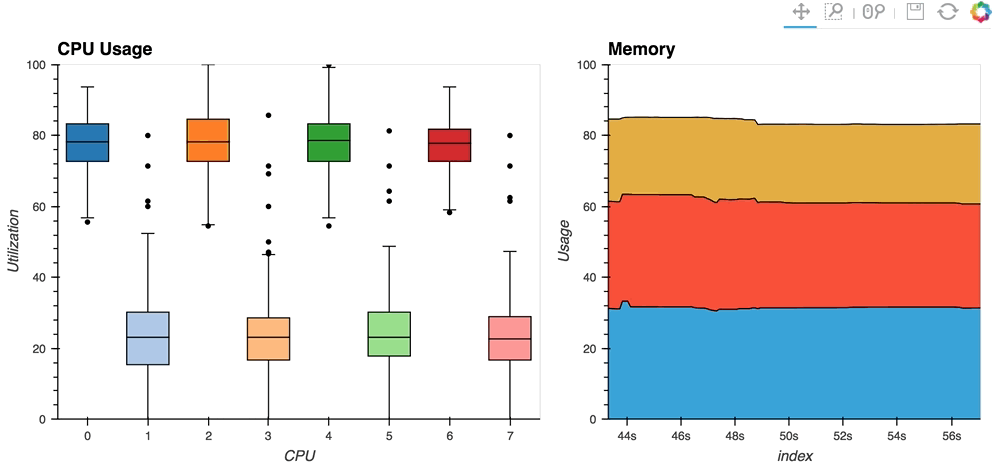Streaming Data¶

“流数据”是连续生成的数据，通常由某些外部源（如远程网站，测量设备或模拟器）生成。这种数据在金融时间序列，Web服务器日志，科学应用程序和许多其他情况下很常见。我们已经了解了如何在[实时数据]（06-Live _Data.ipynb）用户指南中显示可调用的任何数据输出，我们还看到了如何使用HoloViews流系统在用户指南中推送事件部分[响应事件]（11-响应_到Events.ipynb）和[自定义交互]（12-Custom Interactivity.ipynb）。

In [ ]:
import time
import numpy as np
import pandas as pd
import holoviews as hv

from holoviews.streams import Pipe, Buffer

import streamz
import streamz.dataframe

hv.extension('bokeh')

Pipe¶

Pipe允许将数据推送到DynamicMap回调中以更改可视化，就像[响应事件]（./ 11-Responding to Events.ipynb）用户指南中的流一样用于将更改推送到控制可视化的元数据。可以使用Pipe来推送任何类型的数据，并使其可用于DynamicMap回调。由于所有Element类型接受各种形式的data，我们可以使用Pipe通过DynamicMap将数据直接推送到Element的构造函数。

In [ ]:
pipe = Pipe([])
vector_dmap = hv.DynamicMap(hv.VectorField, streams=[pipe])
vector_dmap.redim.range(x=(-1, 1), y=(-1, 1))

In [ ]:
x,y  = np.mgrid[-10:11,-10:11] * 0.1
sine_rings  = np.sin(x**2+y**2)*np.pi+np.pi
exp_falloff = 1/np.exp((x**2+y**2)/8)

for i in np.linspace(0, 1, 25):
time.sleep(0.1)
pipe.send([x,y,sine_rings*i, exp_falloff])

Buffer¶

一个简单的例子：布朗运动¶

In [ ]:
example = pd.DataFrame({'x': [], 'y': [], 'count': []}, columns=['x', 'y', 'count'])
dfstream = Buffer(example, length=100, index=False)
curve_dmap = hv.DynamicMap(hv.Curve, streams=[dfstream])
point_dmap = hv.DynamicMap(hv.Points, streams=[dfstream])

In [ ]:
%%opts Points [color_index='count', xaxis=None, yaxis=None] (line_color='black', size=5)
%%opts Curve (line_width=1, color='black')
curve_dmap * point_dmap

In [ ]:
def gen_brownian():
x, y, count = 0, 0, 0
while True:
x += np.random.randn()
y += np.random.randn()
count += 1
yield pd.DataFrame([(x, y, count)], columns=['x', 'y', 'count'])

brownian = gen_brownian()
for i in range(200):
dfstream.send(next(brownian))

In [ ]:
#dfstream.clear()

使用Streamz库¶

将streamz.Stream和Pipe一起使用¶

1. 声明一个streamz.Stream和一个Pipe对象，并将它们连接到一个我们可以推送数据的管道中。
2. 使用20的sliding_window，它将首先等待20组流更新累积。此时，对于每个后续更新，它将应用pd.concat将最近的20个更新组合到一个新的数据帧中。
3. 使用streamz.Stream上的sink方法来send得到20个更新为Pipe的集合。
4. 声明一个DynamicMap，它采用连接的DataFrames的滑动窗口，并使用Scatter元素显示它。
5. 用“计数”对“散点图”点进行着色并设置范围，然后显示：
In [ ]:
point_source = streamz.Stream()
pipe = Pipe(data=[])
point_source.sliding_window(20).map(pd.concat).sink(pipe.send) # Connect streamz to the Pipe
scatter_dmap = hv.DynamicMap(hv.Scatter, streams=[pipe])

In [ ]:
%%opts Scatter [color_index='count', bgcolor='black']
scatter_dmap.redim.range(y=(-4, 4))

In [ ]:
for i in range(100):
df = pd.DataFrame({'x': np.random.rand(100), 'y': np.random.randn(100), 'count': i},
columns=['x', 'y', 'count'])
point_source.emit(df)

使用StreamingDataFrame和StreamingSeries¶

streamz库（版本0.2）提供了StreamingDataFrameStreamingSeries，它是一种轻松处理表格数据实时源的有效方法。这使得它非常适合使用Buffer。 （在版本0.3streamz已经重命名了类来删除Streaming，所以这里的代码需要更新以用于后来的streamz版本。）使用StreamingDataFrame我们可以轻松传输数据，应用累积和滚动统计等计算，然后使用HoloViews可视化数据。

streamz.dataframe模块提供了一个Random实用程序，它生成一个StreamingDataFrame，它以指定的间隔发出一定频率的随机数据。 example属性让我们可以看到我们可以期待的数据的结构和dtypes：

In [ ]:
simple_sdf = streamz.dataframe.Random(freq='10ms', interval='100ms')
print(simple_sdf.index)
simple_sdf.example.dtypes

In [ ]:
%%opts Curve [width=500 show_grid=True]
sdf1 = (simple_sdf-0.5).cumsum()
hv.DynamicMap(hv.Curve, streams=[Buffer(sdf1.x)])

Random StreamingDataFrame将异步发出事件，向前驱动可视化，直到它被显式停止，我们可以通过调用stop方法来做。

In [ ]:
simple_sdf.stop()

使用StreamingDataFrame API¶

In [ ]:
source_df = streamz.dataframe.Random(freq='5ms', interval='100ms')
sdf2 = (source_df-0.5).cumsum()
sdf2.tail()
In [ ]:
raw       = hv.DynamicMap(hv.Curve, streams=[Buffer(sdf2.x)]).relabel('raw')
smooth    = hv.DynamicMap(hv.Curve, streams=[Buffer(sdf2.x.rolling('500ms').mean())]).relabel('smooth')

raw * smooth.options(width=900,height=350)
In [ ]:
source_df.stop()

对流数据的操作¶

In [ ]:
hist_source = streamz.dataframe.Random(freq='5ms', interval='100ms')
sdf4 = (hist_source-0.5).cumsum()

dmap = hv.DynamicMap(hv.Dataset, streams=[Buffer(sdf4.x, length=500)])
hv.operation.histogram(dmap, dimension='x')
In [ ]:
hist_source.stop()

In [ ]:
%%opts RGB [width=600]
from bokeh.palettes import Blues8
large_source = streamz.dataframe.Random(freq='100us', interval='200ms')
sdf5 = (large_source-0.5).cumsum()

dmap = hv.DynamicMap(hv.Curve, streams=[Buffer(sdf5.x, length=1000000)])
datashade(dmap, streams=[hv.streams.PlotSize], normalization='linear', cmap=Blues8)
In [ ]:
large_source.stop()

In [ ]:
%%opts Curve [width=600]
from tornado.ioloop import PeriodicCallback

count = 0
buffer = Buffer(np.zeros((0, 2)), length=50)

def f():
global count
count += 1
buffer.send(np.array([[count, np.random.rand()]]))

cb = PeriodicCallback(f, 100)
cb.start()

hv.DynamicMap(hv.Curve, streams=[buffer]).redim.range(y=(0, 1))
In [ ]:
cb.stop()

真实的例子¶

• [streaming psutil]（http://holoviews.org/gallery/apps/bokeh/stream psutil.html）散景应用程序是一个这样的例子，使用psutil显示CPU和内存信息library（用pip install psutilconda install psutil安装）